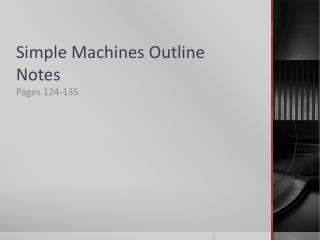DownloadDownload PresentationSimple Machines Outline Notes

# Simple Machines Outline Notes

Télécharger la présentation## Simple Machines Outline Notes

- - - - - - - - - - - - - - - - - - - - - - - - - - - E N D - - - - - - - - - - - - - - - - - - - - - - - - - - -
##### Presentation Transcript

1. Simple Machines Outline Notes Pages 124-135

2. There are 6 basic kinds of simple machines: the inclined plane, the wedge, the screw, the lever, the wheel and axle, and the pulley.

3. 1. Inclined Plane • Inclined plane: flat, sloped surface • Example: ramp

4. A. How It Works • Allows you to exert you input force over a longer distance

5. B. Mechanical Advantage • You can determine the ideal mechanical advantage of an inclined plane by dividing the length of the incline by its height. • Ideal mechanical advantage = length of incline ÷ height of incline

6. 2. Wedge • Wedge: device that is thick at one end and tapers to a thin edge at the other end. • Examples: zipper, knife

7. A. How It Works • Input forces exerted on a wedge causes the output forces to push the wedge into the object.

8. B. Mechanical Advantage • The ideal mechanical advantage of a wedge is determined by dividing the length of the wedge by its width. • The longer and thinner a wedge is, the greater its mechanical advantage.

9. 3. Screws • Screw: inclined plane wrapped around a cylinder • Examples: threads in a jar lid, bolt

10. A. How It Works • Threads of a screw act like an inclined place to increase the distance over which you exert the input force

11. B. Mechanical Advantage • The ideal mechanical advantage of a screw is the length around the threads divided by the length of the screw.

12. 4. Levers • Lever: rigid bar that is free to pivot or rotate on a fixed point • Fulcrum: fixed point that a lever pivots around

13. A. How It Works • When you push down, you exert an input force on the handle which pivots on the fulcrum.

14. B. Mechanical Advantage • The ideal mechanical advantage of a lever is determined by dividing the distance from the fulcrum to the input force by the distance from the fulcrum to the output force. • Ideal mechanical advantage = distance from fulcrum to input force ÷ distance from fulcrum to output force

15. C. Different Types of Levers • 1st class: always change the direction of the input force • 2nd class: increase force but do not change the direction of the input force • 3rd class: increase distance but do not increase the direction of the input force

16. 5. Wheel and Axle • Wheel and axle: simple machine made of 2 circular objects fastened together that rotate about a common axis • Examples: screw driver, doorknob

17. A. How It Works • Increases to your force but you must exert your force over a long distance.

18. B. Mechanical Advantage • You can find the ideal mechanical advantage of a wheel and axle by dividing the radius of the wheel by the radius of the axle. • Mechanical advantage = radius of wheel ÷ radius of axis

19. 6. Pulley • Pulley: simple machine made of a grooved wheel with a rope or cable wrapped around it

20. A. How It Works • You use a pulley by pulling on one end of the rope. • Pulleys can decrease the amount of input force needed to lift an object or it can change the direction of your input force.

21. B. Types of Pulleys • There are 3 types of pulleys: a fixed pulley (attached to a structure), a moveable pulley (attached to the object you are moving), and a block and tackle (combination of a fixed an a moveable pulley). • The ideal mechanical advantage of a pulley is equal to the number of sections of rope that support the object.

22. 7. Simple Machines in the Body

23. A. Living Levers • Most of the machines in your body are levers that consist of bones and muscles.

24. B. Working Wedges • Your teeth are wedges

25. 8. Compound Machines • Compound machine: machine that utilizes 2 or more simple machines • The ideal mechanical advantage of a compound machine is the product of the individual ideal mechanical advantages of the simple machines that make it up.